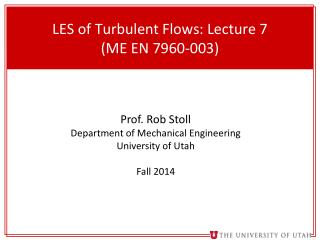Download PresentationLES of Turbulent Flows : Lecture 7 (ME EN 7960-003)# LES of Turbulent Flows : Lecture 7 (ME EN 7960-003) - PowerPoint PPT Presentation

Download Presentation##### LES of Turbulent Flows : Lecture 7 (ME EN 7960-003)

Download Policy: Content on the Website is provided to you AS IS for your information and personal use and may not be sold / licensed / shared on other websites without getting consent from its author. While downloading, if for some reason you are not able to download a presentation, the publisher may have deleted the file from their server.

- - - - - - - - - - - - - - - - - - - - - - - - - - - E N D - - - - - - - - - - - - - - - - - - - - - - - - - - -
##### Presentation Transcript

1. LES of Turbulent Flows: Lecture 7(ME EN 7960-003) Prof. Rob Stoll Department of Mechanical Engineering University of Utah Fall 2014

2. Turbulence modeling (alternative strategies) • So far our discussion of turbulence modeling has centered around separating the flow into resolved and SFSs using a low-pass filtering operation with the goal of reducing the # of degrees of freedom in our numerical solution. • This is not the only way to accomplish complexity reduction in a turbulent flow. Here we briefly review a few other methods • Coherent Vortex Simulations (CVS): Farge and Schneider, Flow Turb. Comb. 2001 • The turbulent flow field is decomposed into coherent and random components using either a continuous or orthonormal wavelet filter (see the next page for a very brief review of wavelets) • In the original formulation the separation between coherent and random motions is assumed to be complete with the random part mimicking viscous dissipation. • Goldstein and Vasilyev (Phys of Fluids, 2004) introduced “stochastic coherent adaptive LES”, a variation on CVS • they use the CVS wavelet decomposition but do not assume that the wavelet filter completely eliminates all the coherent motions from the SFSs => the SFS components themselves contain coherent and random components.

3. Wavelet Decomposition (a brief overview) • The Coherent Vortex Simulations (CVS) method uses wavelet decomposition. • Here is a brief overview of wavelets. For a more detailed view see: • -Daubechies, 1992 (most recent printing is 2006) • -Mallat, 2009 (3rd edition) • -Farge, Ann. Rev. Fluid Mech., 1992 (specific to turbulence research) • First: Windowed Fourier Transforms (or Gabor transform) • recall: • a windowed Fourier transform can be expressed as: • -where s is the position over a localized region • -the windowed transform is our convolution • with a filter function in Fourier space f(s)g(s)1 f(x) g(s)1

4. Wavelet decomposition (a brief overview) • Wavelets offer an optimal space frequency decomposition, in 1D: • where Ψ is the basis function (sometimes called the “Mother Wavelet”), b translates the basis function (location) and a scales the basis function (dilatation). • A few different types of Wavelets exist, the main general classes (similar to Fourier) are: • -continuous transforms (what we wrote above) • -discrete transforms • Redundant discrete systems • Orthonormal systems (this is used in CVS)

5. Wavelet decomposition (a brief overview) • Common Properties of Wavelets (Burrus et al., 1998): • A wavelet transform is a set of building blocks to construct or represent a signal (function). It is a 2D expansion set (usually a basis) for a 1D signal. • A wavelet expansion gives a time-frequency localization of a signal • The calculation of coefficients can be done efficiently • Wavelet systems are generated from a single scaling function (ie wavelet) by simple scaling and translation • Most useful wavelet systems satisfy the multiresolution condition. If the basic expansion signals (the wavelets) are made half as wide and translated in steps half as wide, they will represent a larger class of signals exactly or give a better approximation of any signal. • The lower resolution coefficients can be calculated from the higher resolution coefficients by a tree-structured algorithm. • Example of a common wavelet (see listed refs for others): • HaarWavelet (Haar, 1910): Basic square wave Different a, b values

6. Wavelet decomposition (a brief overview) • How does wavelet decomposition break down a signal in space and time? Fourier basis real space Wavelet basis Windowed Fourier basis wavenumber wavenumber wavenumber space space wavenumber space space

7. Wavelet decomposition (a brief overview) • Example: Haar Wavelet decomposition • The signal is reconstructed by combining si and di at the desired level (s, ss, sss, etc). • 1st level – si and di • 2nd level – ssi, ddi, di • … Wavelet “tree” decomposition Wavelet “tree” decomposition x1 x2 x3 x4x5 x6 x7 x8 x1 x2 x3 x4x5 x6 x7 x8 s1 s2 s3 s4 d1 d2 d3 d4 ss1 ss2 dd1 dd2 sss1 ddd1 For the Haar wavelet s1=(x1+x2)/2½ For the Haar wavelet d1=(x2-x1)/2½

8. Turbulence modeling (alternative strategies) • Filtered Density Functions (FDF): Colucci et al., (Phys. of Fluids, 1998) • -In this method, the evolution of the filtered probability density functions is solved for (i.e., we solve for the evolution of the SFS general moments) • -Similar to general PDF transport methods 1st introduced by Lundgren (Phys. of Fluid, 1969) and outlined in detail in Pope chapter 12. • -Many applications use FDF for scalars in turbulent reacting flows while traditional (low-pass filtered N-S) equations are solved for momentum. For a more extensive discussion see Fox, 2002. • -This type of method is often employed for LES with Lagrangian particle models and for chemically reactive flows. In Lagrangian particle models it leads to a form of theLangevin equations for SFS particle evolution and in chemically reactive flows it has the advantage that the reactions occur in closed form • -We will return to these type of methods later in the class when we discuss combing LES with particle models.